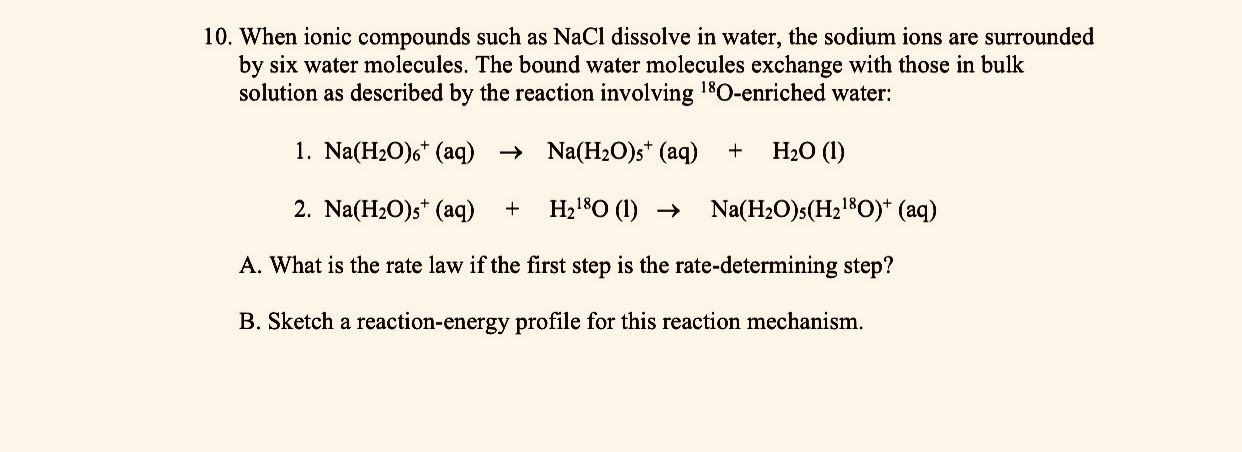# When Ionic compounds such as NaCl dissolves in water, the sodium ions are surrounded by six water molecules. The bound water molecules exchange with those in bulk solution as described by the reaction involving 18O-enriched water: 1. Na(H2O)6+ (aq) → Na(H2O)5+ (aq) + H2O (l) 2. Na(H2O)5+ (aq) + H218O (l) → Na(H2O)5(H218O)+ (aq) A. What is the rate law if the first step is the rate-determining step? B. Sketch a reaction-energy profile for this reaction mechansim.